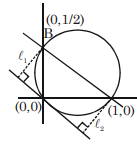# The straight line x + 2y = 1 meets the coordinate axes at A and B.`
Question:

The straight line $x+2 y=1$ meets the coordinate axes at A and B. A circle is drawn through A, $B$ and the origin. Then the sum of perpendicular distances from $A$ and $B$ on the tangent to the circle at the origin is :

1. $\frac{\sqrt{5}}{4}$

2. $\frac{\sqrt{5}}{2}$

3. $2 \sqrt{5}$

4. $4 \sqrt{5}$

Correct Option: , 2

Solution:Equation of circle

$(x-1)(x-0)+(y-0)\left(y-\frac{1}{2}\right)=0$

$\Rightarrow x^{2}+y^{2}-x-\frac{y}{2}=0$

Equation of tangent of origin is $2 \mathrm{x}+\mathrm{y}=0$

$\ell_{1}+\ell_{2}=\frac{2}{\sqrt{5}}+\frac{1}{2 \sqrt{5}}$

$=\frac{4+1}{2 \sqrt{5}}=\frac{\sqrt{5}}{2}$